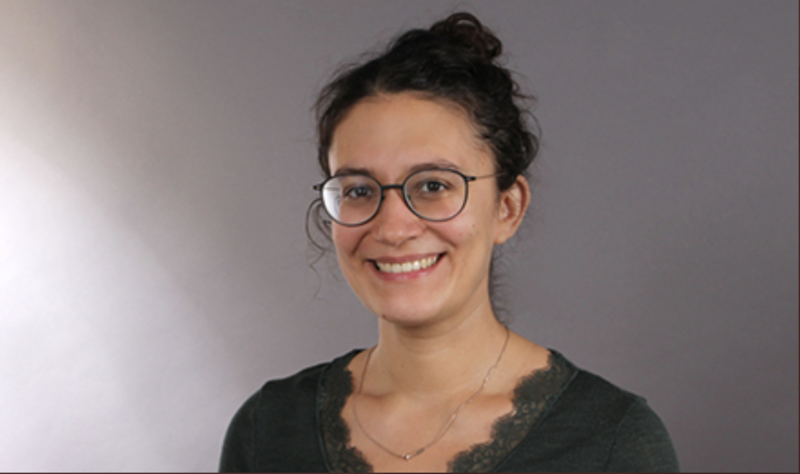# BIDSA SEMINAR SERIES "Optimal permutation estimation in crowd sourcing"Room 3-E4-SR03
-

Alexandra Carpentier, Institute of Mathematics, Potsdam University

Consider a crowd sourcing problem where we have n experts and d tasks. The average ability of each expert for each task is stored in an unknown matrix M, which is only observed in noise and incompletely. The aim is then to rank the experts by ability, based on these partial and noisy observations. We make no (semi) parametric assumptions, but rather assume that a shape constraint is satisfied by the matrix M: namely either that (1) the tasks can be perfectly ranked by difficulty, as well as the experts by competence up to a permutation, or (2) that the experts can be perfectly ranked by competence up to a permutation.
We provide a minimax-optimal and computationally feasible method for this problem, based on hierarchical clustering, PCA, and exchange of informations among the clusters. We prove in particular - in the case where d > n - that the problem of estimating the expert ranking is significantly easier than the problem of estimating the matrix M.
This is based on joint works with Emmanuel Pilliat and Nicolas Verzelen.